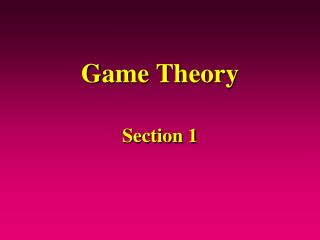# Game Theory - PowerPoint PPT PresentationDownload PresentationGame Theory

Game Theory
Download Presentation## Game Theory

- - - - - - - - - - - - - - - - - - - - - - - - - - - E N D - - - - - - - - - - - - - - - - - - - - - - - - - - -
##### Presentation Transcript

1. Game Theory Section 1

2. Agenda • What is game theory? • Exercise: convergence to equilibrium • Key terms and definitions • Formal theory: what Leo has to say about it • How do you do it (using formal theory) • Application: Alliance Formation • Final thoughts

3. What Defines Game Theory? Formal Analysis of Strategic Settings • Mathematically precise • List of players • Complete description of allowable moves • Description of information • Specification of how actions cause outcomes • Specification of players’ preferences

4. Exercise: Moving to Equilibrium Player 2 A Game in Normal Form Avg payoff for playing with prob distribution p, given others play strategies with prob distribution q Expected Value:

5. Dominant Strategy One best strategy no matter what your opponent does Weakly dominant versus strongly (strictly dominant) strategies Mixed Strategy Moves are chosen randomly from the set of pure strategies Every simultaneous move game has a Nash equilibrium in mixed strategies Three or Four Important Concepts Beliefs: Probability distribution over strategies of other players Nash equilibrium: When each player is playing a best response to the strategies of the others

6. Key Terms and Definitions • Normal form and extensive form • Nodes (initial, terminal) • Information sets • Strategy: complete contingent plan • One for every information set • Equilibrium: a stability concept • Welfare • Pareto optimality: no free lunch

7. Formal Theory: What’s it good for? “We understand only what we make” --Leo Strauss We have absolutely certain or scientific knowledge only of those subjects of which we are the causes… The construction must be conscious construction; it is impossible to know a scientific truth without knowing at the same time that we have made it. The world of our constructs is therefore the desired island that is exempt from the flux of blind and aimless causation.

8. Applying Formal Theory • Goal (research question) • Story (model) • Formalization (abstraction) • Analysis (manipulation) • Solution (definition of equilibrium) • Translation (external and internal validity) Game Theory is just one of several formal modeling approaches General Equilibrium: interactions among many (infinite) agents, where any one agent’s actions have no effect on other agents.

9. Example: Alliance Formation • Goal: • Gain insight into dynamics of power and motivation • What motivates countries to form alliances? • Story: • Setting: 3 countries fighting a war • Each has power (military capability), which is positively associated with probability of winning • Each is rational and has its own interests at heart Example taken from Altfield and Bueno de Mesquita 1979

10. Formalization • States are identified as (A, B, C) • Their capabilities are (a,b,c) • If B helps A, probability that A wins is (a + b)/(a + b + c) = PBA • If B helps C, probability that C wins is (b + c)/(a + b + c) = PBC • Amount that B values a victory by A is UBA • Cost to B of helping A is KBA • Cost of neutrality is zero • B vs. nature: B moves, then either A or C wins

11. Analysis: Whither Alliances? • Adopt the perspective of a player: B • B’s utility from an alliance with A = PBA(UBA) + (1-PBA)(UBC) - KBA • B’s utility from an alliance with C = PBC(UBC) + (1-PBC)(UBA) - KBC (1) (2) • What if equation 1 > equation 2? • What if equation 2 > equation 1?

12. Solution: Equilibrium What is the point at which B is indifferent? PBA(UBA) + (1-PBA)(UBC) - KBA )= PBC(UBC) + (1-PBC)(UBA) - KBC ) Simplifies to... (PBA +PBC -1)(UBA -UBC) = (KBA -KBC) Simplifies to... [b/(a + b + c)] (UBA -UBC) = (KBA -KBC)

13. Translation: Validity • What is the point at which B is indifferent? [b/(a + b + c)] (UBA -UBC) = (KBA -KBC) • [b/(a + b + c)] = resources B can contribute • (UBA -UBC) = B’s motivation for A vs. C • (KBA -KBC) = B’s costs for A vs. C The decision to help in a dispute depends on one’s ability to influence the outcome, one’s level of motivation, and the costliness of getting involved • Calibration, comparative statics, case studies are all ways of assessing model validity

14. Final thoughts • Given B is indifferent to allying when • [b/(a + b + c)] (UBA -UBC) = (KBA -KBC), and • [b/(a + b + c)] = resources B can contribute • (UBA -UBC) = B’s motivation for A vs. C • (KBA -KBC) = B’s costs for A vs. C • Equilibrium, perturbed: • If the left-hand side is > the right-hand side then B would rather partner with A • What real-world events might prompt this?